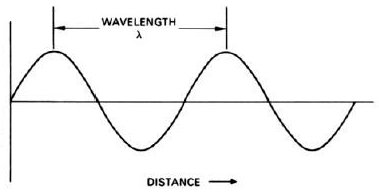# Acoustic waveThis article ws checked by pedagogue, but later was changed.Checked version of the article can be found here.

## Acoustic Waves

Acoustic waves are mechanical and longitudinal waves (same direction of vibration as the direction of propagation) that result from an oscillation of pressure that travels through a solid, liquid or gas in a wave pattern. These waves show numerous characteristics including wavelength, frequency, period and amplitude. Acoustic waves are perceived by the ear as sound.

### Wavelength

Distance between two consecutive points with the same spacing from the equilibrium positions and the same oscillatory movement. It depends on the media in which the wave is propagating. The SI unit is meter (m).### Frequency

Frequency is the number of oscillations, or waves, per unit of time. Sound waves with higher frequencies have higher pitches than those with lower frequencies. It depends only on the frequency of oscillation of the emitting source. The SI unit is hertz (Hz).### Period

The time interval between the emission of two pulses is given by the period, which is reciprocal to the frequency. This characteristic only depends on the period of oscillation of the emitting source. The SI unit is the second (s).### Amplitude

Amplitude is the maximum deviation in the oscillation relatively to the equilibrium position. The greater the amplitude of an acoustic wave, the louder the sound. It depends on the amplitude of the emitting source and on the propagation medium. The SI unit is the meter (m).### Speed of Sound

Acoustic waves travel with the same speed as sound. Sound speed is the distance travelled by the sound during a given time and is dependent on temperature and pressure conditions. At Normal Temperature (15 °C) and Pressure (NTP) conditions sound speed has a value of 340 ms-1.